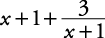## Polynomials

A polynomial consists of two or more terms. For example, x + y, y2x2, and x2 + 3 x + 5 y2 are all polynomials. A binomial is a polynomial that consists of exactly two terms. For example, x + y is a binomial. A trinomial is a polynomial that consists of exactly three terms. For example, y2 + 9 y + 8 is a trinomial.

Polynomials usually are arranged in one of two ways. Ascending order is basically when the power of a term increases for each succeeding term. For example, x + x2 + x3 or 5 x + 2 x2 – 3 x3 + x5 are arranged in ascending order. Descending order is basically when the power of a term decreases for each succeeding term. For example, x3 + x2 + x or 2 x4 + 3 x2 + 7 x are arranged in descending order. Descending order is more commonly used.

To add or subtract polynomials, just arrange like terms in columns and then add or subtract. (Or simply add or subtract like terms when rearrangement is not necessary.)

##### Example 1

Do the indicated arithmetic.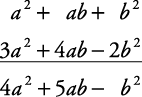2.3. Subtract the polynomials.4.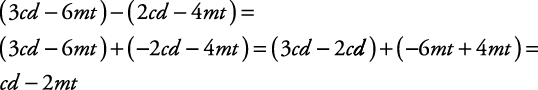5.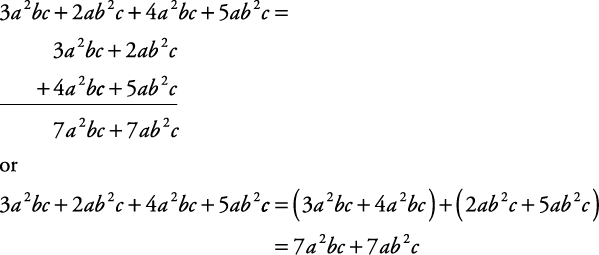#### Multiplying polynomials

To multiply polynomials, multiply each term in one polynomial by each term in the other polynomial. Then simplify if necessary.

##### Example 2

Multiply.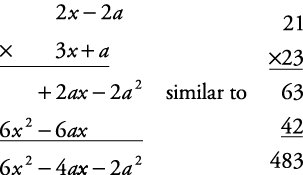Or you may want to use the “ F.O.I.L.” method with binomials. F.O.I.L. means First terms, Outside terms, Inside terms, Last terms. Then simplify if necessary.

##### Example 3

Multiply.

(3 x + a)(2 x – 2 a) =

Multiply first terms from each quantity.Now outside terms.Now inside terms.Finally last terms.Now simplify.

6 x2 – 6 ax + 2 ax – 2 a2 = 6 x2 – 4 ax – 2 a2

##### Example 4

Multiply.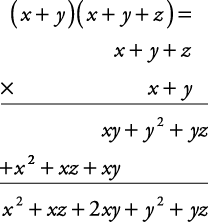This operation also can be done using the distributive property.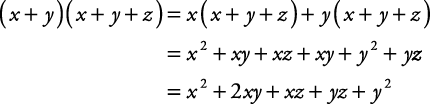#### Dividing polynomials by monomials

To divide a polynomial by a monomial, just divide each term in the polynomial by the monomial.

##### Example 5

Divide.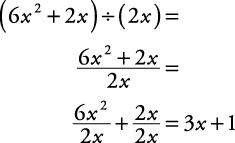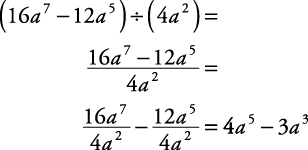#### Dividing polynomials by polynomials

To divide a polynomial by a polynomial, make sure both are in descending order; then use long division. ( Remember: Divide by the first term, multiply, subtract, bring down.)

##### Example 6

Divide 4 a2 + 18 a + 8 by a + 4.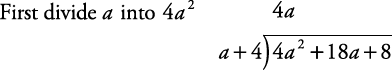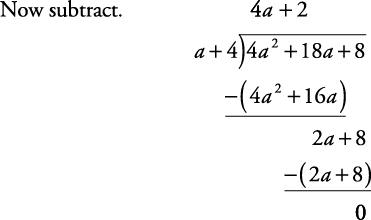##### Example 7

Divide.

1.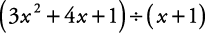2.First change to descending order: x2 + 2 x + 1. Then divide.3.Note: When terms are missing, be sure to leave proper room between terms.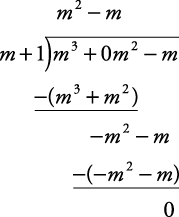4.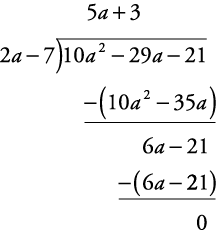5.This answer can be rewritten as